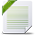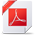# Global Data Science Forum

View Only

## linear mixed models - estimating R square#### Karolina ZiembowiczFri May 20, 2022 05:41 AM#### Shad GriffinMon May 23, 2022 10:15 AM• #### 1.  linear mixed models - estimating R square

Posted Fri May 20, 2022 05:41 AM
Hello,
I have the following problem with my data.
I have two significant interaction terms in the model which accounts for the random effect of a group (small group interactions). However, R square, calculated as:
(random effects residuals - full model residuals)/ random effect residuals has horribly low values (.003 for the model with one interaction term, .01 for the model with two interaction terms). When I add these terms in a regular regression, both the total r-square and r-square changes are much higher. how can it be?

I understand that regular regression does not separate random effects from fixed effects, however, the estimates are quite high.

Maybe my calculation of R square is not right? Any help?

Cheers,
Karolina

------------------------------
Karolina Ziembowicz
------------------------------

Attachment(s)results.pdf   69 KB 1 version

• #### 2.  RE: linear mixed models - estimating R square

Posted Mon May 23, 2022 10:15 AM

Just want to make sure I understand.  So you the random effects are not nearly as strong in terms of R-squared that you'd expect?  When you add random effects to a model with fixed effects, they do little to increase the R-Squared?

------------------------------